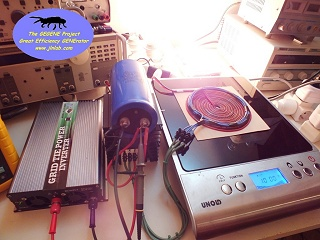TEST #12 : New measurements with the flat bifilar coil v1.1 and the new induction cooker
created on december 28, 2012 - JLN Labs - last update january 16, 2013
All informations and diagrams are published freely (freeware) and are intended for a private use and a non commercial use.
Toutes les informations et schémas sont publiés gratuitement ( freeware ) et sont destinés à un usage personnel et non commercial

Cliquez ici pour la version FRANCAISE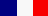January 15, 2013 - TEST #12 : Here is a new test with the flat bifilar coil v1.1 and the new induction cooker manufactured by "Rosenstein & Söhne" and with the reference NC-3050. In this test, the output power has been limited to the level 5/10 i.e. at the middle power (about 1200 W).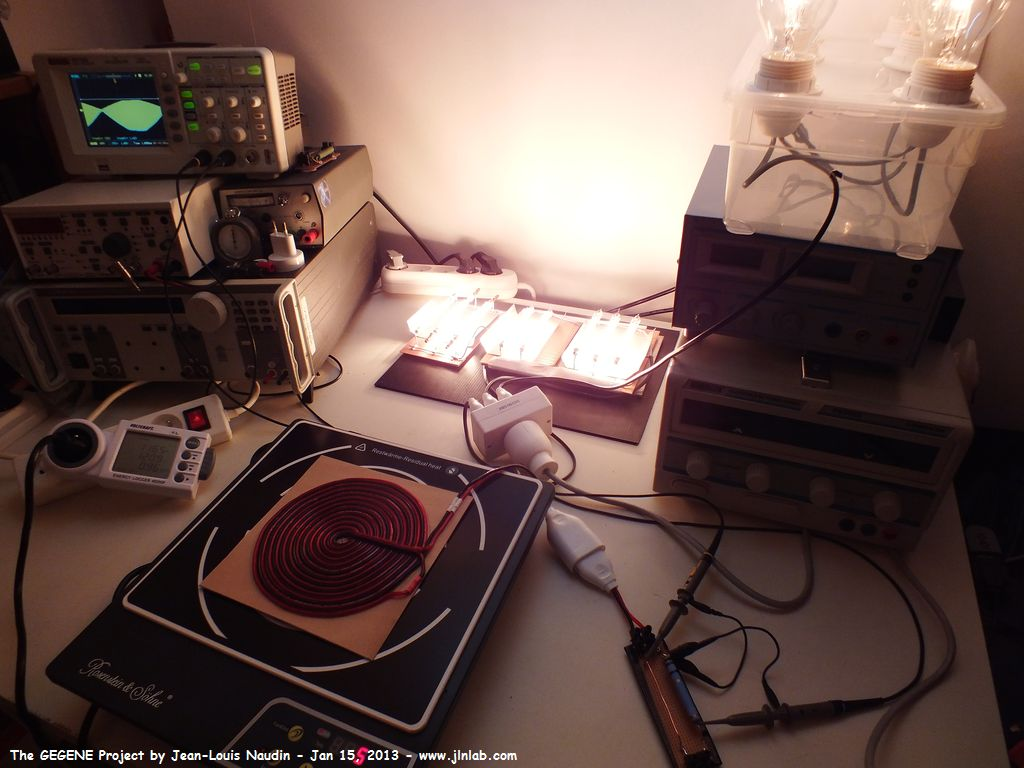This model of induction cooker is very appreciable because I don't need to use a ferromagnetic sheet to start the ignition of the halogen lamps.

In this test, I have used this setup below of halogen lamps as loads :

• 1 box of 6 halogenes lamps of 105 W
• 2 sets of halogen which contains :
• 1 set of halogens tubes : ((2 x 240 W) + (1 x 400 W)) + (2 x 500 W)
• 1 set of halogens tubes : (1 x 240 W) + (2 x 400 W)

The TOTAL POWER of the LOAD connected at the OUTPUT of the GEGENE is 3550 Watts

TEST #12 RESULTS :

To measure the voltage and the current at the output for powering the halogen lamps, the two scope probes are set to X10 and are connected to the flat bifilar coil output. The probe Ch1 is used to measure the voltage accross the flat bifilar coil output and the probe Ch2 is used to measure the current i.e. the voltage accross a 0.1 Ohm non inductive resistor Mundorf MR10 10W. Then the datas are sent to a datasheet to compute true RMS values and the efficiency. The electrical power input of the induction cooker is measured with a Wattmeter Energy Logger 4000F directly connected on the power grid.The True RMS values of the voltages on Ch1 and Ch2 are computed in real time by the Rigol digital oscilloscope ...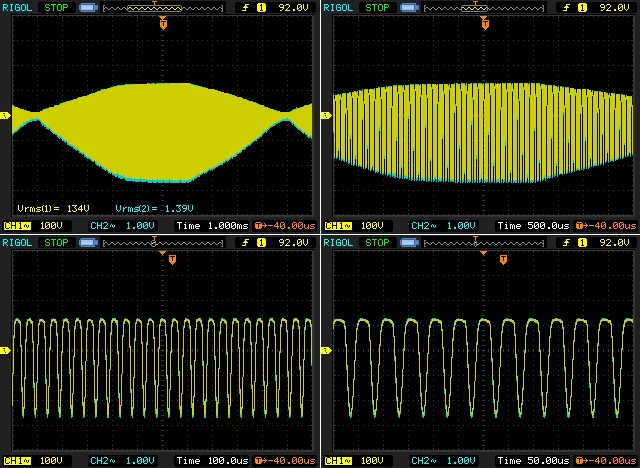The electrical power for the induction cooker is measured with a Wattmeter Energy Logger 4000F directly connected on the power grid :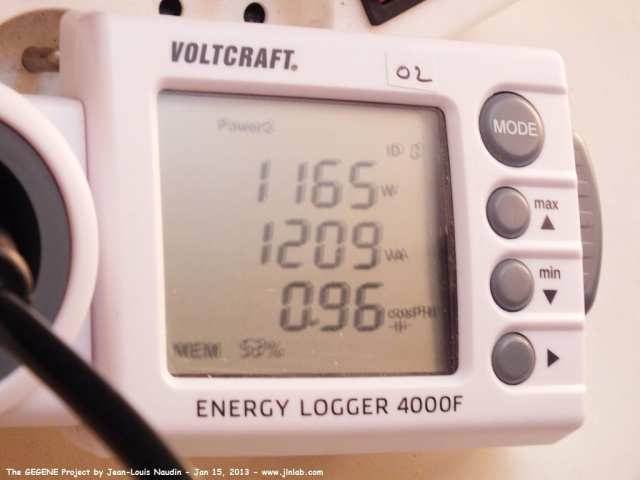The Wattmeter measures 1165 Watt at the INPUT of the induction cooker.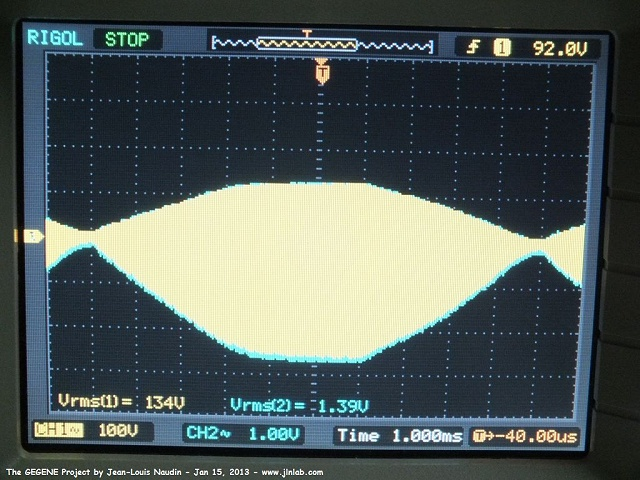Above the True RMS voltage measured by the Rigol digital oscilloscope.

So as to get a good accuracy of the measured values for the mathematical computation, the sampling rate has been set to 20.00 MSa/s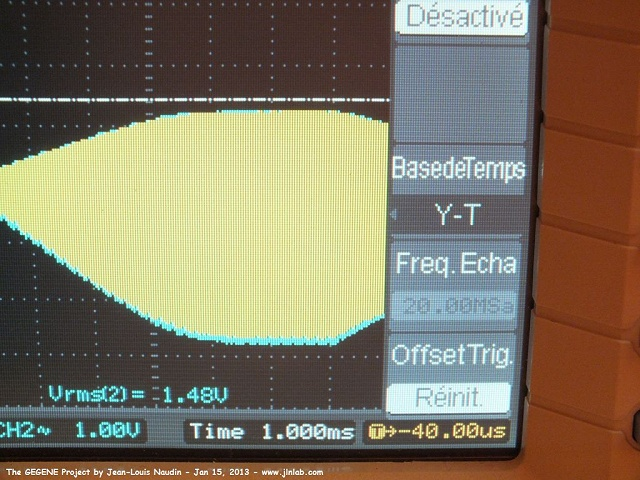Below, the scope datas computed with GNU Octave 3.2.4 (freeware and full compatible version of MATLAB)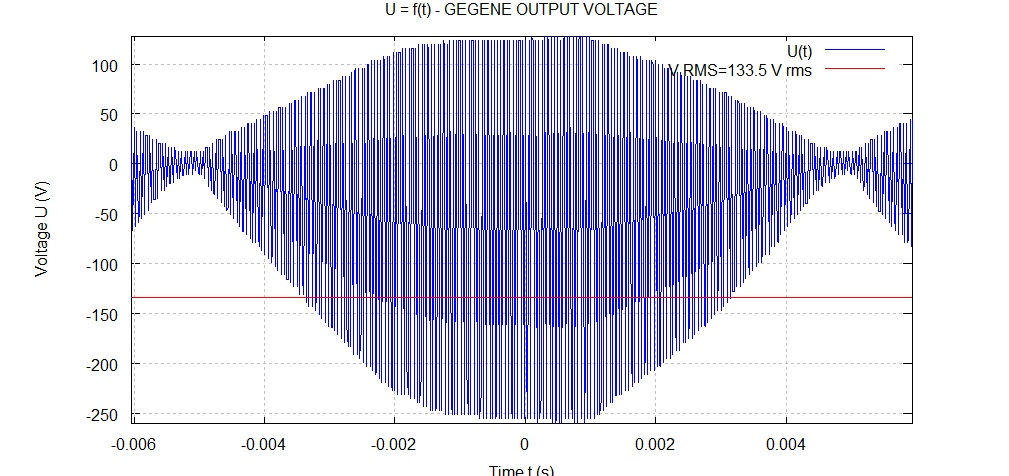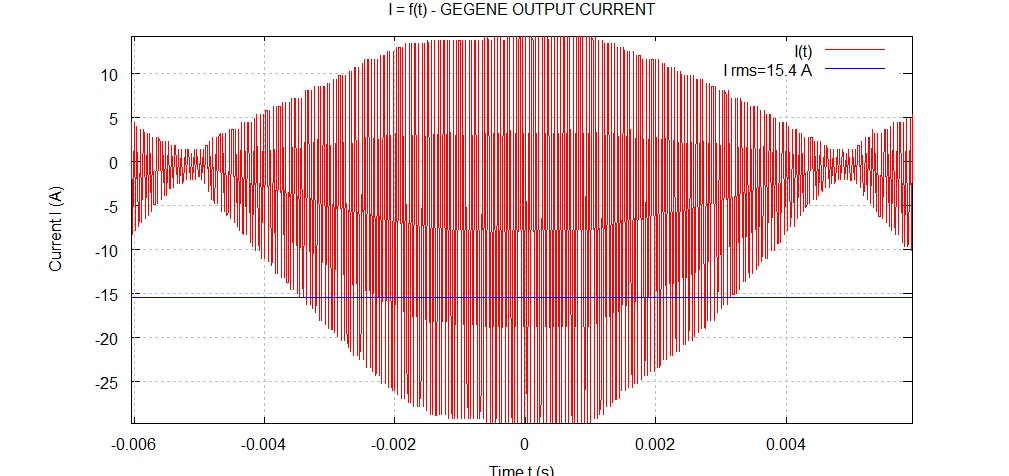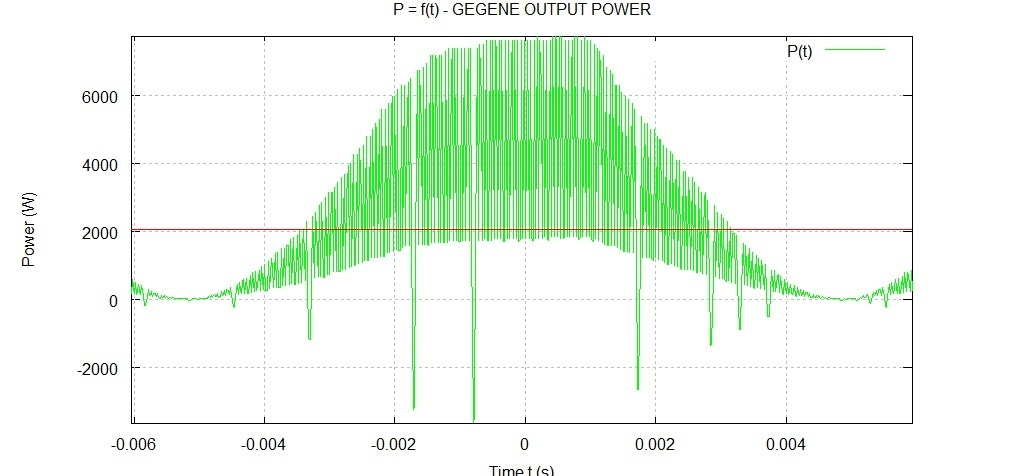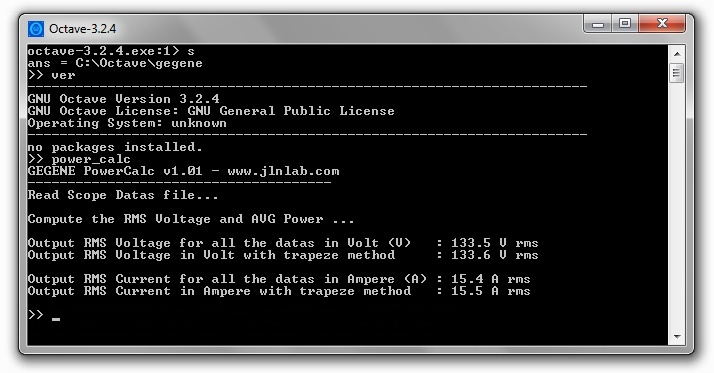Here is my software source used to compute the datas coming from the Rigol digital oscilloscope for Octave 3.2.4 or Matlab R7 or more

 % GEGENE Project - RMS Voltage, Current and Power calculator % by Jean-Louis Naudin - January 15, 2013 % www.jlnlab.com % % This program runs on OCTAVE Version 3.2.4 or MATHLAB r7.0 or more % GNU General Public Licence - this is a freeware % % v1.01 : add the trapeze method calculation % clear all; close all; clc r=0.09; % Current probe resistance value in Ohm fprintf('GEGENE PowerCalc v1.01 - www.jlnlab.com\n'); fprintf('--------------------------------------\nRead Scope Datas file...\n\n'); datas = dlmread('NewFile0.csv', ',',2,0); % read the datas scope file % Below a sample of the CSV datas from the Rigol digital oscilloscope % X,CH1,CH2, % Second,Volt,Volt, % -3.72e-03,8.40e+01,7.60e-01, % -3.70e-03,-3.20e+01,-3.60e-01, % -3.68e-03,-2.80e+01,-2.80e-01, l=length(datas); % numbers of sample t=datas(1:l,1); % t: time base u1=datas(1:l,2); % ch1: voltage output u2=datas(1:l,3); % ch2: voltage accros the current probe % % compute the RMS Voltage % fprintf('Compute the RMS Voltage and AVG Power ...\n\n'); %VTG_rms=norm(u1)/sqrt(length(u1)); VTG_rms = sqrt(sum(u1.*conj(u1))/size(u1,1)); veff=sqrt(trapz(t,u1.*u1)/(t(l)-t(1))); vrms = zeros(l,1); vrms = vrms + VTG_rms; figure(1); clf; plot(t,u1,'-b',t,vrms,'-r',t,-vrms,'-r'); xlabel('Time t (s)'); ylabel('Voltage U (V)'); title('U = f(t) - GEGENE OUTPUT VOLTAGE'); axis([t(1) t(l) min(u1) max(u1)]); disp(['Output RMS Voltage for all the datas in Volt (V) : ',sprintf('%0.1f',VTG_rms),' V rms']); disp(['Output RMS Voltage in Volt with trapeze method : ',sprintf('%0.1f',veff),' V rms']); grid on legend('U(t)',['V RMS=',sprintf('%0.1f',VTG_rms),' V rms']); % % compute the RMS Current % ip2=u2/r; %CUR_rms=norm(u2/r)/sqrt(length(u2)); CUR_rms = sqrt(sum(u2/r.*conj(u2/r))/size(u2/r,1)); ceff=sqrt(trapz(t,ip2.*ip2)/(t(l)-t(1))); crms = zeros(l,1); crms = crms + CUR_rms; figure(2); clf; plot(t,ip2,'-r',t,crms,'-b',t,-crms,'-b'); xlabel('Time t (s)'); ylabel('Current I (A)'); title('I = f(t) - GEGENE OUTPUT CURRENT'); axis([t(1) t(l) min(ip2) max(ip2)]); disp(['Output RMS Current for all the datas in Ampere (A) : ',sprintf('%0.1f',CUR_rms),' A rms']); disp(['Output RMS Current in Ampere with trapeze method : ',sprintf('%0.1f',ceff),' A rms']); grid on legend('I(t)',['I rms=',sprintf('%0.1f',CUR_rms),' A']); % % compute the Average Power % pp=u1.*u2/r; Pavg = VTG_rms * CUR_rms; pmoy=trapz(t,pp)/(t(l)-t(1)); pwravg = zeros(l,1); pwravg = pwravg + Pavg; figure(3); clf; plot(t,pp,'-g',t,pwravg,'-r'); xlabel('Time t (s)'); ylabel('Power (W)'); title('P = f(t) - GEGENE OUTPUT POWER'); axis([t(1) t(l) min(pp) max(pp)]); disp(['---> OUTPUT AVG Power (VTG_rms * CUR_rms): ',sprintf('%0.1f',Pavg),' Watt ']); disp(['---> OUTPUT AVG Power (Trapeze method) : ',sprintf('%0.1f',pmoy),' Watts']); grid on legend('U(t)',['AVG PWR =',sprintf('%0.1f',Pavg),' W ']);

Under Octave launch power_calc

NEXT TEST :

Stay tuned,

Email: jnaudin509@aol.com